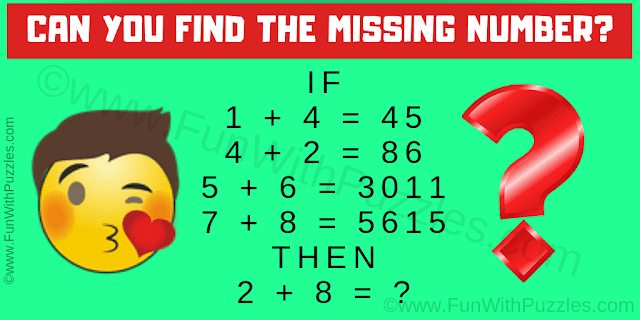This Logic Math Question is for school going students. It will test your logical reasoning and mathematical skills. In this math logic question, you are shown some number equation. Break the logical reasoning used in these number equations and solve the last equation. Can you find the missing number?Can you solve this Logic Math Question?
Answer of this "Logic Math Question", can be viewed by clicking on the answer button.